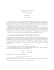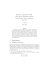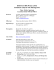# AP Calculus AB - Worksheet 19

## Transcription

AP Calculus AB - Worksheet 19
```AP Calculus AB - Worksheet 19
Tangent and Normal Lines (Power Rule)
Learn: Tangent and Normal Lines to a Curve
Recall: Derivative = slope of the Tangent line at that point’s x-coordinate
Example:
For each of the following:
a) Sketch a graph - USE GRAPH PAPER!!
b) Find the slope of the tangent line at the given point.
c) Find the equations of the tangent line at the given point. Sketch the line.
d) Find the equation of the normal to the curve at the given point. Sketch the line.
( )
1. y = x 2 − 3, 2,1
2.
3.
4.
Find the equations of the tangent and normal lines to the curve at the given x-value.
5.
6.
7.
8. Find the points on the curve
where the tangent is horizontal.
9. For what values of x does the graph of
10. Show that the curve
have a horizontal tangent?
has no tangent line with slope 4.
11. Find an equation of the tangent line to the curve
that is parallel to the line
12. Find equations of both lines that are tangent to the curve
13. Find a parabola with equation
through the point
.
( x + Δx )
lim
3
14. Evaluate
Δx→0
(
that has slope 4 at
) (
− 2 x + Δx − x 3 − 2x
Δx
).
.
and are parallel to the line
, slope -8 at
, and passes
.
```

### Alvin Jin Math 16A: Worksheet 2 9/4/14 Today, we'll be trying to get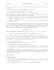### Name: __________________________________________________________________ Period: ___________________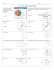### Math 083 Sample Exam 3 A Given: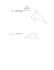### Math 120 Sample questions for Test 2, with answers### Tuesday, October 7th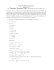### MAT 272 Calculus and Analytic Geometry III 10/11/2011 Sample solutions for test 2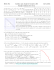### CIVIL SURVEYING SAMPLE EXAMS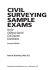### MATH 121, Calculus I — Sample Exam I (Fall 2013) Name: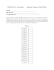### Tangent and normal lines.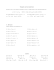### Solutions to the 2015 AP Calculus AB Free Response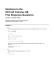### Manual Tangent Fjord Dock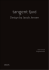### AP Calculus 3.1 Worksheet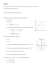### G.C.2 Wkst 3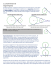### Assoc. Prof. Cornel Pintea Geometry for First Year students Tutorial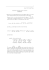### Gaussian Curvature ∗ Yan-Bin Jia Nov 4, 2014